Pandas Time Series Examples: DatetimeIndex, PeriodIndex and TimedeltaIndex

# Pandas Time Series Examples: DatetimeIndex, PeriodIndex and TimedeltaIndex

Last updated:

View all code in this jupyter notebook

For more examples on how to manipulate date and time values in pandas dataframes, see Pandas Dataframe Examples: Manipulating Date and Time

## Use existing date column as index

If your dataframe already has a date column, you can use use it as an index, of type DatetimeIndex:

import pandas as pd

# this is the original dataframe
df = pd.DataFrame({
'name':[
'john','mary','peter','jeff','bill'
],
'date_of_birth':[
'2000-01-01', '1999-12-20', '2000-11-01', '1995-02-25', '1992-06-30',
],
})

print(df.index)
# RangeIndex(start=0, stop=5, step=1)

# convert the column (it's a string) to datetime type
datetime_series = pd.to_datetime(df['date_of_birth'])

# create datetime index passing the datetime series
datetime_index = pd.DatetimeIndex(datetime_series.values)

df2=df.set_index(datetime_index)

# we don't need the column anymore
df2.drop('date_of_birth',axis=1,inplace=True)

print(df2.index)
# DatetimeIndex(['2000-01-01', '1999-12-20', '2000-11-01', '1995-02-25',
#    '1992-06-30'], dtype='datetime64[ns]', freq=None)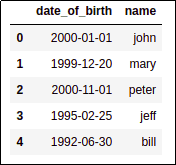BEFORE: If you don't specify an
index when creating a dataframe,
by default it's a RangeIndex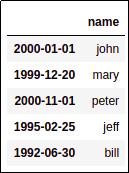AFTER: After setting the index to
the date column, the index is now
of type DatetimeIndex

## Add rows for empty periods

import pandas as pd

df = pd.DataFrame({
'name':[
'john','mary','peter','jeff','bill'
],
'day_born':[
'1988-12-28', '1988-12-25', '1988-12-24', '1988-12-26',  '1988-12-30'
],
})
df

df.index
# RangeIndex(start=0, stop=5, step=1)

# build a datetime index from the date column
datetime_series = pd.to_datetime(df['day_born'])
datetime_index = pd.DatetimeIndex(datetime_series.values)

# replace the original index with the new one
df3=df.set_index(datetime_index)

# we don't need the column anymore
df3.drop('day_born',axis=1,inplace=True)

# IMPORTANT! we can only add rows for missing periods
# if the dataframe is SORTED by the index
df3.sort_index(inplace=True)

df3.index
# DatetimeIndex(['1988-12-24', '1988-12-25', '1988-12-26', '1988-12-28',
#               '1988-12-30'],
#              dtype='datetime64[ns]', freq=None)

# 'D' stands for 'DAYS'
df4=df3.asfreq('D')

df4.index
# DatetimeIndex(['1988-12-24', '1988-12-25', '1988-12-26', '1988-12-27',
#                '1988-12-28', '1988-12-29', '1988-12-30'],
#              dtype='datetime64[ns]', freq='D')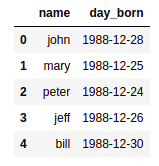df: original dataframe. Note that
there is no data for 27th
and 29th of December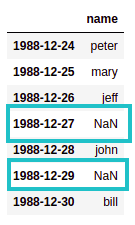df4: after calling asfreq(), extra
rows (in blue) have been

## Create lag columns using shift

template: .shift(<number_of_periods>, <offset_alias>) where the alias is one of 'D' for days, 'W' for weeks, etc.

In many cases you want to use values for previous dates as features in order to train classifiers, analyze data, etc.

from datetime import date

import numpy as np
import pandas as pd

# create a dummy dataset
df = pd.DataFrame(
index=pd.to_datetime([date(2019,1,d) for d in range(1,11)])
)

# D minus 1 = current day minus 1

# create columns for 2 days before as well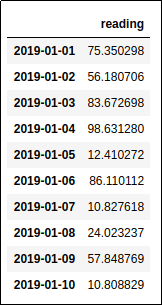This dataframe has one reading of each day from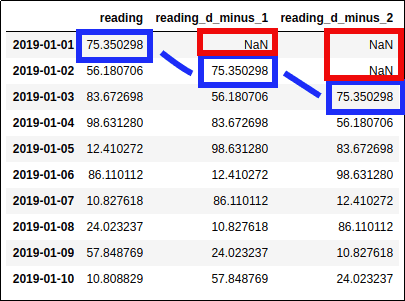Two lagged columns were added to the right
Note that there are NaNs (red) when